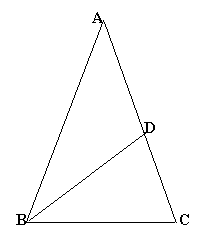#### You may also like### Gold Again

Without using a calculator, computer or tables find the exact values of cos36cos72 and also cos36 - cos72.### Pythagorean Golden Means

Show that the arithmetic mean, geometric mean and harmonic mean of a and b can be the lengths of the sides of a right-angles triangle if and only if a = bx^3, where x is the Golden Ratio.### Golden Powers

You add 1 to the golden ratio to get its square. How do you find higher powers?

# Golden Triangle

##### Age 16 to 18 Challenge Level:

 The three triangles are all isoceles so $\angle BDC = \angle ACB = \angle ABC.$$\angle BDC = \angle BAC + \angle DBA = 2 \angle BAC.$

$\angle BCD + \angle ABC + \angle BAC = \angle BDC + \angle BDC + \angle BAC = 180^o.$

$2 \angle BAC + 2 \angle BAC + \angle BAC = 180^o$
$5 \angle BAC = 180^o$,
$\angle BAC = \angle DBA = 36^o$,
$\angle BDA = 108^o$ ,
$\angle ABC = \angle ACB = \angle BDC = 72^o$
and $\angle CBD = 36^o.$

The triangles $ABC$ and $BDC$ have lengths $AB = AC = p$ and $BC = BD = DA = q$ and the angles are $36^o , 72^o$ and $72^o$ so they are similar triangles. Taking the ratio of corresponding sides $AC/BC = BC/DC$ : $$\frac{p}{q} = \frac{q}{p-q}$$ So $p^2 -pq-q^2 = 0$ and dividing by $q^2$ gives the quadratic equation $$(p/q)^2 -(p/q)-1=0$$ which has the solutions $(1 \pm \sqrt 5) /2$. We don't want the negative root for such a ratio as it would make no sense. Hence $p/q = (\sqrt{5} + 1)/2$. Similarly $q/p = (\sqrt 5 - 1)/2$.

Triangles $ABC$ and $BDC$ are similar and the ratio of areas is the square of the ratio of corresponding sides. So area of
$BDC : ABC$ $= [(\sqrt 5 - 1)^2/2 ] : 1$
$= (6 -2\sqrt 5 )/4 : 1$
$= (3 - \sqrt 5 ) : 2$

So if the area of triangle $ABC$ is $2$ the area of triangle $BDC$ is $3-\sqrt 5$ and the area of triangle $ABD$ is $2 - (3 - \sqrt 5) = \sqrt 5 -1$.